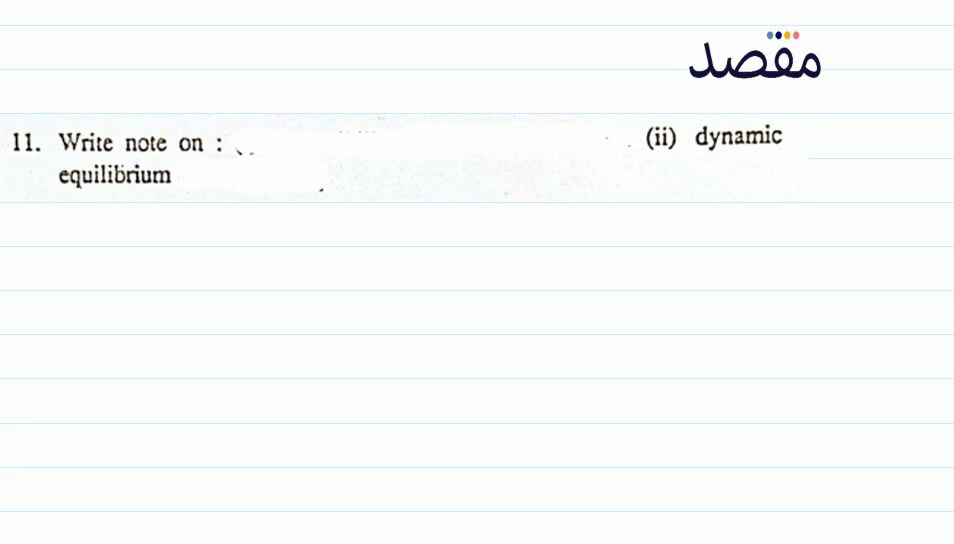# Classes

Change the way you learn with Maqsad's classes. Local examples, engaging animations, and instant video solutions keep you on your toes and make learning fun like never before!

Class 9Class 10First YearSecond Year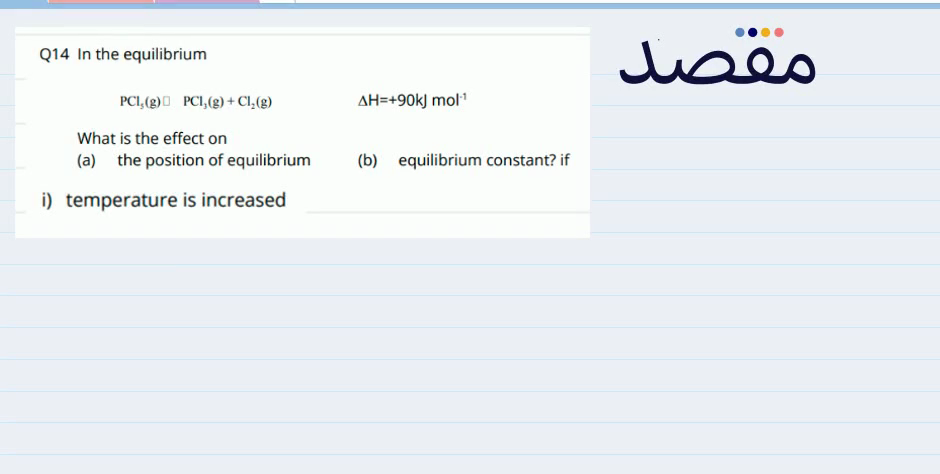### Q14 In the equilibrium$\mathrm{PCl}_{5}(\mathrm{~g}) \square \mathrm{PCl}_{3}(\mathrm{~g})+\mathrm{Cl}_{2}(\mathrm{~g}) \Delta \mathrm{H}=+90 \mathrm{~kJ} \mathrm{~mol}^{-1}$What is the effect on(a) the position of equilibrium(b) equilibrium constant? ifi) temperature is increased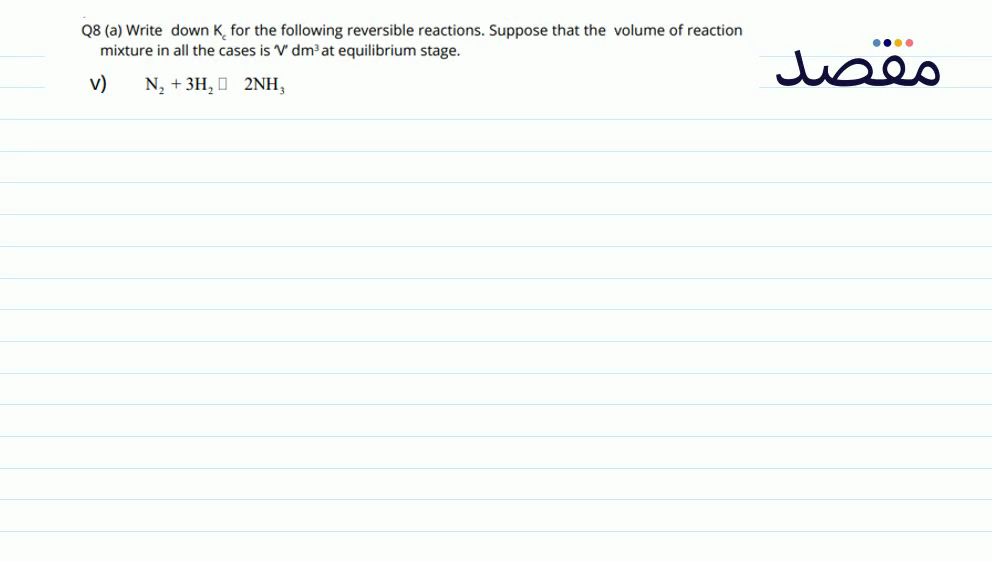### Q8 (a) Write down \mathrm{K}_{c} for the following reversible reactions. Suppose that the volume of reaction mixture in all the cases is V^{\prime \prime} \mathrm{dm}^{3} at equilibrium stage.V) \mathrm{N}_{2}+3 \mathrm{H}_{2} \square 2 \mathrm{NH}_{3}### ii) Which statement about the following equilibrium is correct 2 \mathrm{SO}_{2}(\mathrm{~g})+\mathrm{O}_{2}(\mathrm{~g}) \square 2 \mathrm{SO}_{3}(\mathrm{~g}) \ddot{A} \mathrm{H}=-188.3 \mathrm{~kJ} \mathrm{~mol}{ }^{-1} (a) The value of K_{p} falls with a rise in temperature(b) The value of K_{p} falls with increasing pressure(c) Adding \mathrm{V}_{2} \mathrm{O}_{5} catalyst increase the equilibrium yield of sulphur trioxide(d) The value of K_{0} is equal to K_{\text {c. }} .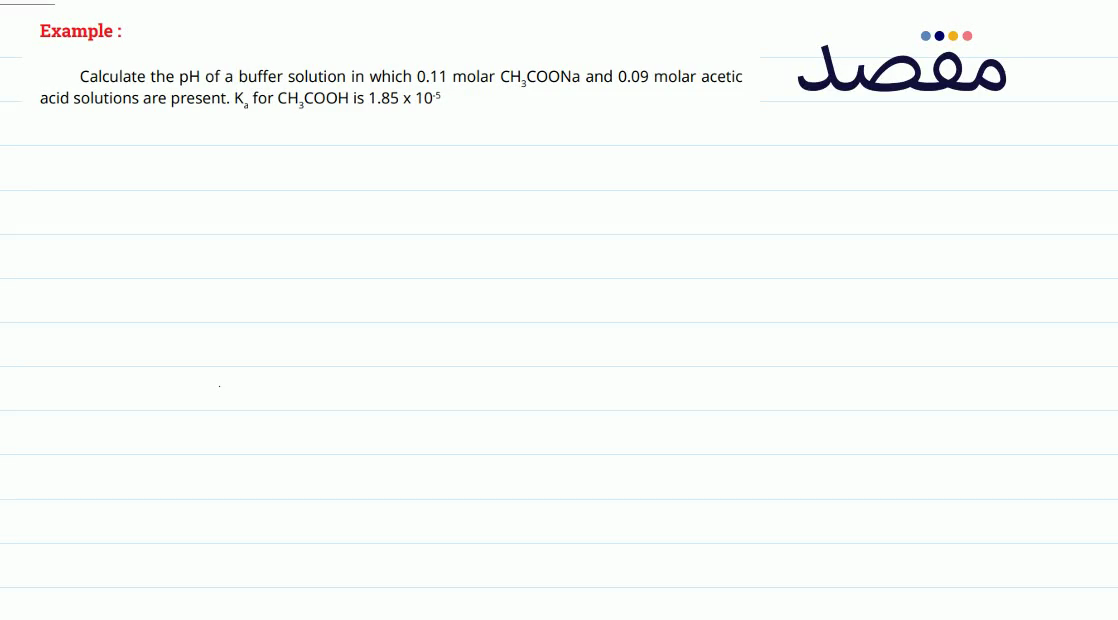### Example:Calculate the \mathrm{pH} of a buffer solution in which 0.11 molar \mathrm{CH}_{3} \mathrm{COONa} and 0.09 molar acetic acid solutions are present. \mathrm{K}_{\mathrm{a}} for \mathrm{CH}_{3} \mathrm{COOH} is 1.85 \times 10^{-5}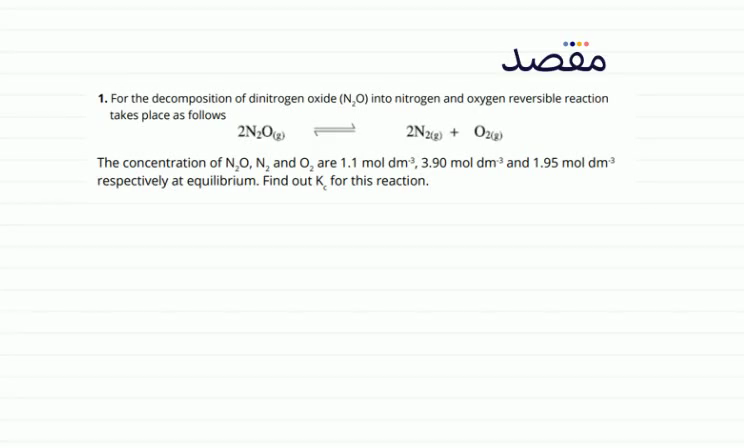### 1. For the decomposition of dinitrogen oxide \left(\mathrm{N}_{2} \mathrm{O}\right) into nitrogen and oxygen reversible reaction takes place as follows$2 \mathrm{~N}_{2} \mathrm{O}_{(\mathrm{g})} \rightleftharpoons 2 \mathrm{~N}_{2(\mathrm{~g})}+\mathrm{O}_{2(\mathrm{~g})}$The concentration of \mathrm{N}_{2} \mathrm{O} \mathrm{N}_{2} and \mathrm{O}_{2} are 1.1 \mathrm{~mol} \mathrm{dm}^{-3} 3.90 \mathrm{~mol} \mathrm{dm}^{-3} and 1.95 \mathrm{~mol} \mathrm{dm}^{-3} respectively at equilibrium. Find out \mathrm{K}_{\mathrm{c}} for this reaction.